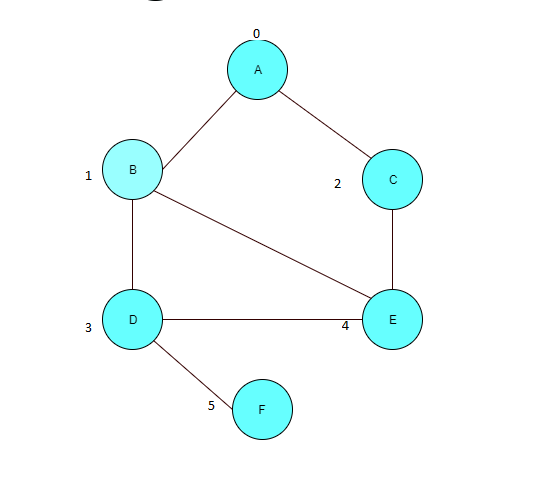Graph representation – How do you represent a graph as an adjacency list?

In this post, we are going to study how to represent a graph as an adjacency list. An adjacency list is an array of the linked list. Each element of the array has a linked list associated with it, which contains the vertices adjacent to the vertex at the index of the array.Observe the above representation of the graph as an adjacency list. Each of the first element determines the vertex in the graph and the linked list shows the elements adjacent to the vertex.

We can also put the data for weights or any other representational data in the vertex for the graph. The time complexity for finding relationships between vertices is O(n). However, this representation saves us on the space that is required to store the graph as compared to adjacency matrix representation

We will represent the graph in a java program as below:

```import java.util.LinkedList;

public class GraphDemo {

public static void main(String[] args) {
Graph graph = new Graph(5);

graph.printGraph();
}

}

class Graph {

// number of vertices
int V;

// initailize graph's vertices with respective linked lists.
Graph(int V) {
this.V = V;
for (int i = 0; i < V; i++) {
}
}

// ads edges to the graph. Takes a graph, a src vertice and destination
// vertice
public void addEdges(int src, int dest) {
// undirected graph
}

public void printGraph() {

for (int i = 0; i < this.V; i++) {
System.out.println("Adjacency list for vertex " + i);
System.out.print("start");
for (Integer j : this.adjArray[i]) {
System.out.print(" -> " + j);
}
System.out.println("\n");
}

}

}```

core java 12 Algorithm 12